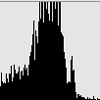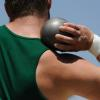#### You may also likeGiven the mean and standard deviation of a set of marks, what is the greatest number of candidates who could have scored 100%?### David and Goliath

Does weight confer an advantage to shot putters?

# Maximum Scattering

##### Age 16 to 18Challenge Level

Ruth from Manchester High School for Girls sent us her work on this problem. Well done, Ruth!

(a) When there are $2$ numbers, their total is $2$. The possibilities for the values of the two numbers can be represented as a straight line $x+y=2$ on a graph. The mean is the point $(1,1)$ in the centre of the line. The standard deviation ($\sigma$) is greatest when the distance between the values and the mean is largest. The endpoints are furthest from the centre so $\sigma$ is largest at the point $(2,0)$ when it is equal to $1$.

(b) When there are $3$ values, their total is $3$. This is represented by a plane. As the numbers are non-negative the only values for the numbers are in a triangle with the corners at $(0,0,3)$, $(0,3,0)$ and $(3,0,0)$. These are the points furthest away from the mean which is at $(1,1,1)$ so are where $\sigma$ is greatest and it is $\sqrt{2}$.
(c) When there are $n$ values, the total is $n$. The region where this is true and they are non-negative is a $n-1$-dimensional shape with $n$ corners which have coordinates $(0,0,...,0,n)$ each with the $n$ in a different position and $n-1$ $0$s. These are furthest from the mean (which is at $(1,1,...,1,1)$) so are where the value of $\sigma$ is greatest. $\sigma$ is the square root of the difference between the square of mean and the mean of the squares. The mean is $1$ so the mean squared is $1$. The squares are $n^{2}$, 0, 0, ... so the total of the squares is $n^{2}$ and their mean is $n$. This makes $\sigma= \sqrt{n-1}$.

In fact, as is explained in the notes , we'd have to do some geometry for (c) to prove that the points are indeed the furthest from the mean. But for this question all we are asked to do is to show that the standard deviation can take a certain value, and Ruth has done this.